# 在r语言中使用GAM（广义相加模型）进行电力负荷时间序列分析

``DT <- as.data.table(read_feather("DT_4_ind"))``

``````DT[, week_num := as.integer(car::recode(week,
"'Monday'='1';'Tuesday'='2';'Wednesday'='3';'Thursday'='4';
'Friday'='5';'Saturday'='6';'Sunday'='7'"))]``````

``````n_type <- unique(DT[, type])
n_date <- unique(DT[, date])
n_weekdays <- unique(DT[, week])
period <- 48``````

``````data_r <- DT[(type == n_type & date %in% n_date[57:70])]

ggplot(data_r, aes(date_time, value)) +
geom_line() +
theme(panel.border = element_blank(),
panel.background = element_blank(),
panel.grid.minor = element_line(colour = "grey90"),
panel.grid.major = element_line(colour = "grey90"),
panel.grid.major.x = element_line(colour = "grey90"),
axis.text = element_text(size = 10),
axis.title = element_text(size = 12, face = "bold")) +
labs(x = "Date", y = "Load (kW)")``````

R语言广义相加模型（GAM）在电力负荷预测中的应用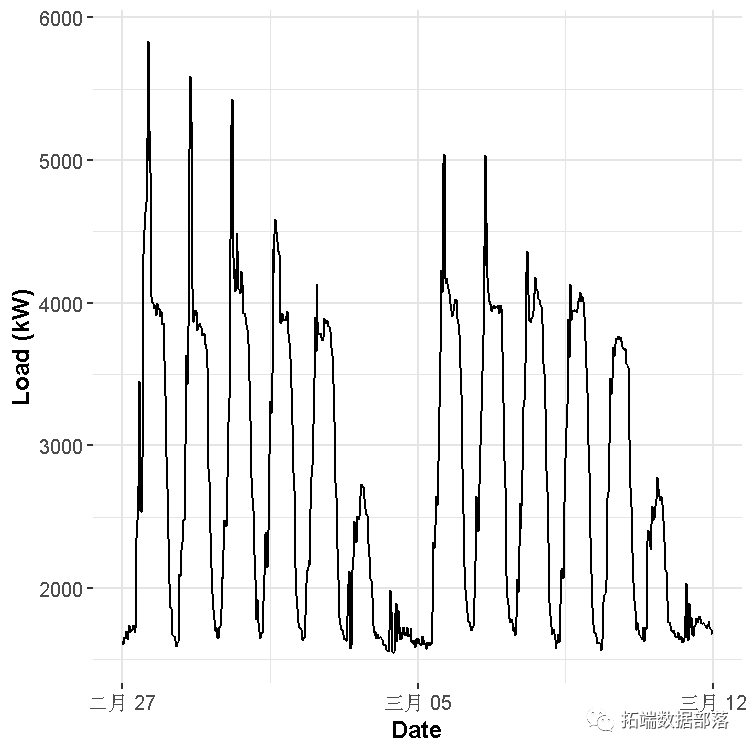​​

``````gam_1 <- gam(Load ~ s(Daily, bs = "cr", k = period) +
s(Weekly, bs = "ps", k = 7),
data = matrix_gam,
family = gaussian)``````

``````layout(matrix(1:2, nrow = 1))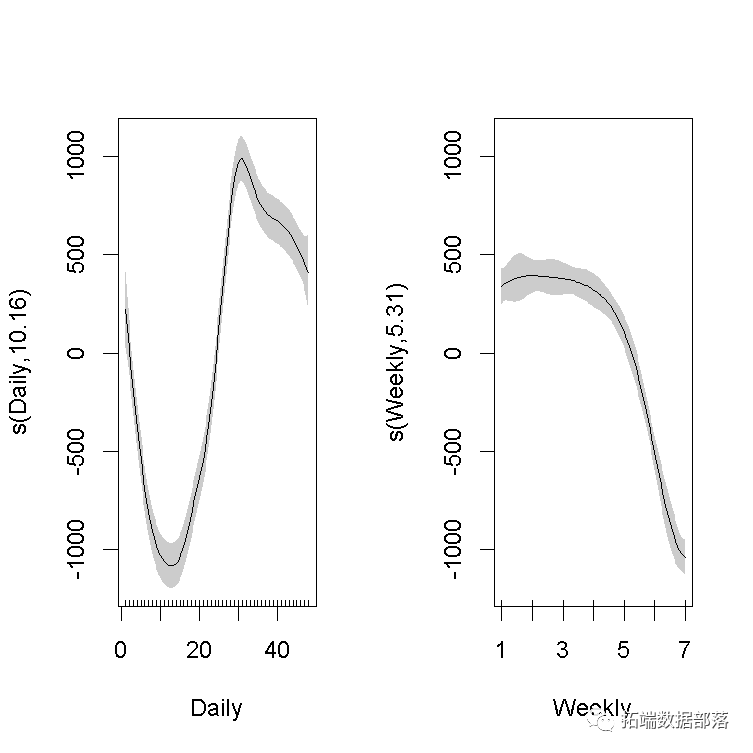​​

``````##
## Family: gaussian
##
## Formula:
## Load ~ s(Daily, bs = "cr", k = period) + s(Weekly, bs = "ps",
##     k = 7)
##
## Parametric coefficients:
##             Estimate Std. Error t value Pr(>|t|)
## (Intercept)  2731.67      18.88   144.7   <2e-16 ***
## ---
## Signif. codes:  0 '***' 0.001 '**' 0.01 '*' 0.05 '.' 0.1 ' ' 1
##
## Approximate significance of smooth terms:
##              edf Ref.df     F p-value
## s(Daily)  10.159 12.688 119.8  <2e-16 ***
## s(Weekly)  5.311  5.758 130.3  <2e-16 ***
## ---
## Signif. codes:  0 '***' 0.001 '**' 0.01 '*' 0.05 '.' 0.1 ' ' 1
##
## R-sq.(adj) =  0.772   Deviance explained = 77.7%
## GCV = 2.4554e+05  Scale est. = 2.3953e+05  n = 672``````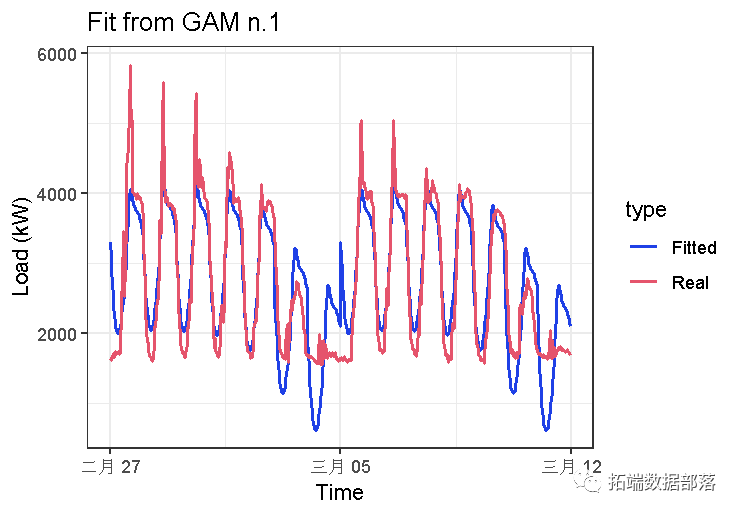​​

``````gam_2 <- gam(Load ~ s(Daily, Weekly),
data = matrix_gam,
family = gaussian)

summary(gam_2)\$r.sq``````
``##  0.9352108``

R方值表明结果要好得多。

``summary(gam_2)\$s.table``
``````##                     edf   Ref.df        F p-value
## s(Daily,Weekly) 28.7008 28.99423 334.4754       0``````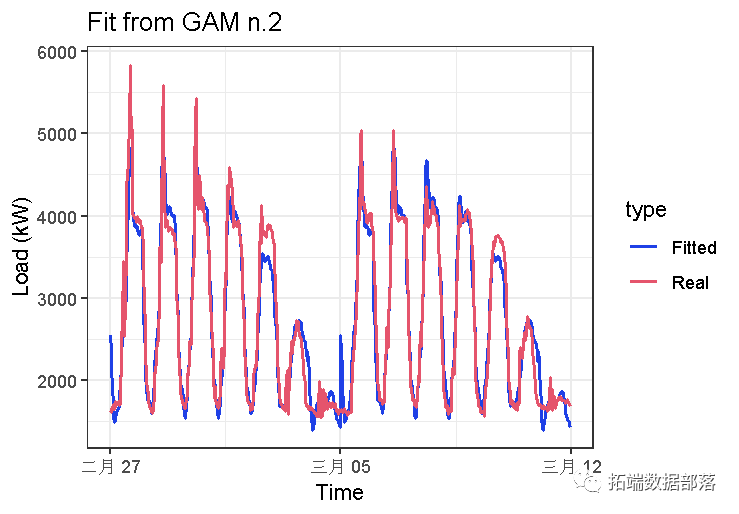​​

``##  0.9268452``

``summary(gam_3)\$s.table``
``````##                       edf   Ref.df        F p-value
## te(Daily,Weekly) 23.65709 23.98741 354.5856       0``````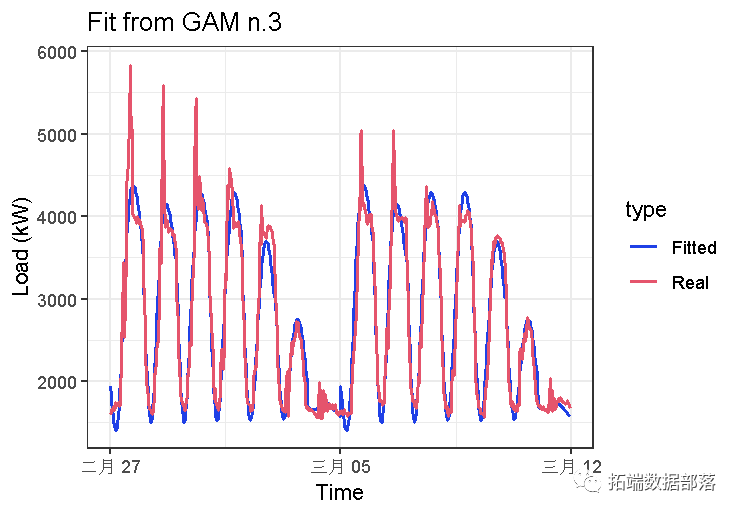​​

# R语言对用电负荷时间序列数据进行K-medoids聚类建模和GAM回归

``##  0.9727604``
``summary(gam_4)\$sp.criterion``
``````##   GCV.Cp
## 34839.46``````
``summary(gam_4)\$s.table``
``````##                       edf   Ref.df        F p-value
## te(Daily,Weekly) 119.4117 149.6528 160.2065       0``````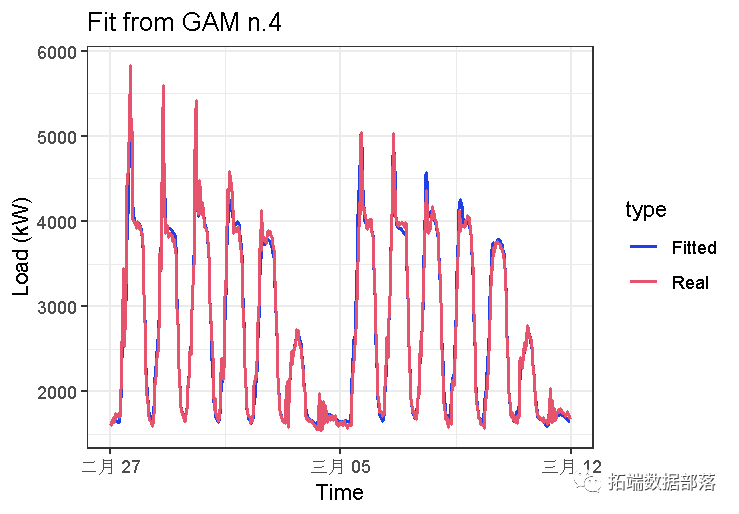​​

``##  0.965618``
``summary(gam_4_fx)\$s.table``
``````##                  edf Ref.df        F       p-value
## te(Daily,Weekly) 335    335 57.25389 5.289648e-199``````

# 我们可以看到R平方比模型`gam_4`低，这是因为我们过度拟合了模型。证明GCV程序（lambda和EDF的估计）工作正常。

``##  0.9717469``
``summary(gam_5)\$sp.criterion``
``````##   GCV.Cp
## 35772.35``````
``summary(gam_5)\$s.table``
``````##                        edf     Ref.df          F p-value
## s(Daily)         22.583649  27.964970  444.19962       0
## s(Weekly)         5.914531   5.995934 1014.72482       0
## ti(Daily,Weekly) 85.310314 110.828814   41.22288       0``````

### 随时关注您喜欢的主题

``##  0.9738273``
``summary(gam_6)\$sp.criterion``
``````##   GCV.Cp
## 32230.68``````
``summary(gam_6)\$s.table``
``````##                       edf   Ref.df        F p-value
## t2(Daily,Weekly) 98.12005 120.2345 86.70754       0``````

``AIC(gam_4, gam_5, gam_6)``
``````##             df      AIC
## gam_4 121.4117 8912.611
## gam_5 115.8085 8932.746
## gam_6 100.1200 8868.628``````

# 我们可以看到的模型的拟合值`gam_4`和`gam_6`非常相似。可以使用软件包的更多可视化和模型诊断功能来比较这两个模型。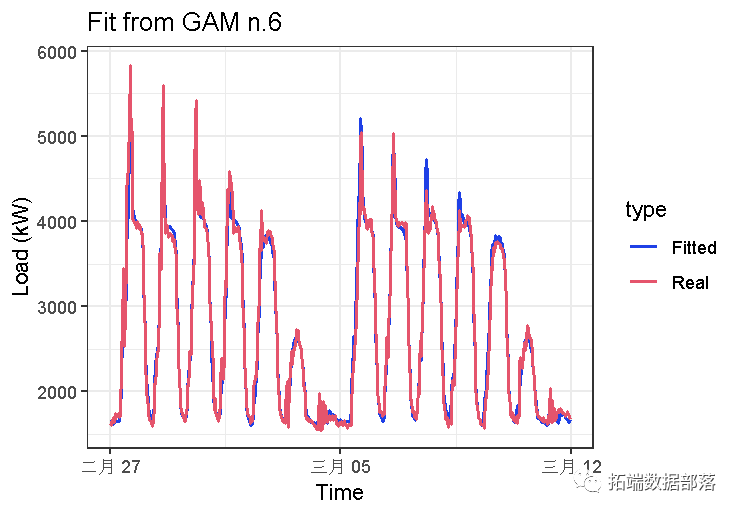​​

``gam.check(gam_4)``

``````##
## Method: GCV   Optimizer: magic
## Smoothing parameter selection converged after 7 iterations.
## The RMS GCV score gradiant at convergence was 0.2833304 .
## The Hessian was positive definite.
## The estimated model rank was 336 (maximum possible: 336)
## Model rank =  336 / 336
##
## Basis dimension (k) checking results. Low p-value (k-index<1) may
## indicate that k is too low, especially if edf is close to k'.
##
##                      k'    edf k-index p-value
## te(Daily,Weekly) 335.00 119.41    1.22       1``````
``gam.check(gam_6)``

``````##
## Method: GCV   Optimizer: magic
## Smoothing parameter selection converged after 9 iterations.
## The RMS GCV score gradiant at convergence was 0.05208856 .
## The Hessian was positive definite.
## The estimated model rank was 336 (maximum possible: 336)
## Model rank =  336 / 336
##
## Basis dimension (k) checking results. Low p-value (k-index<1) may
## indicate that k is too low, especially if edf is close to k'.
##
##                      k'    edf k-index p-value
## t2(Daily,Weekly) 335.00  98.12    1.18       1``````

``````layout(matrix(1:2, nrow = 1))
plot(gam_4, rug = FALSE, se = FALSE, n2 = 80, main = "gam n.4 with te()")
plot(gam_6, rug = FALSE, se = FALSE, n2 = 80, main = "gam n.6 with t2()")``````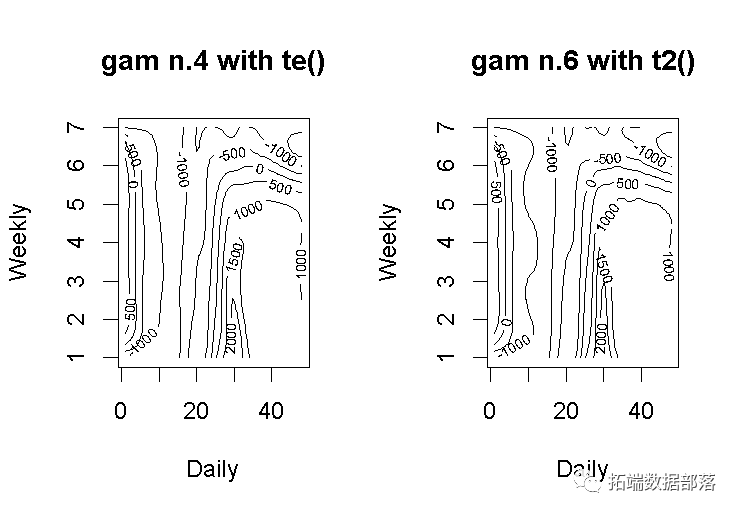​​

该模型`gam_6 `有更多的“波浪形”的轮廓。因此，这意味着它对因变量的拟合度更高，而平滑因子更低。

``````vis.gam(gam_6, n.grid = 50, theta = 35, phi = 32, zlab = "",
ticktype = "detailed", color = "topo", main = "t2(D, W)")``````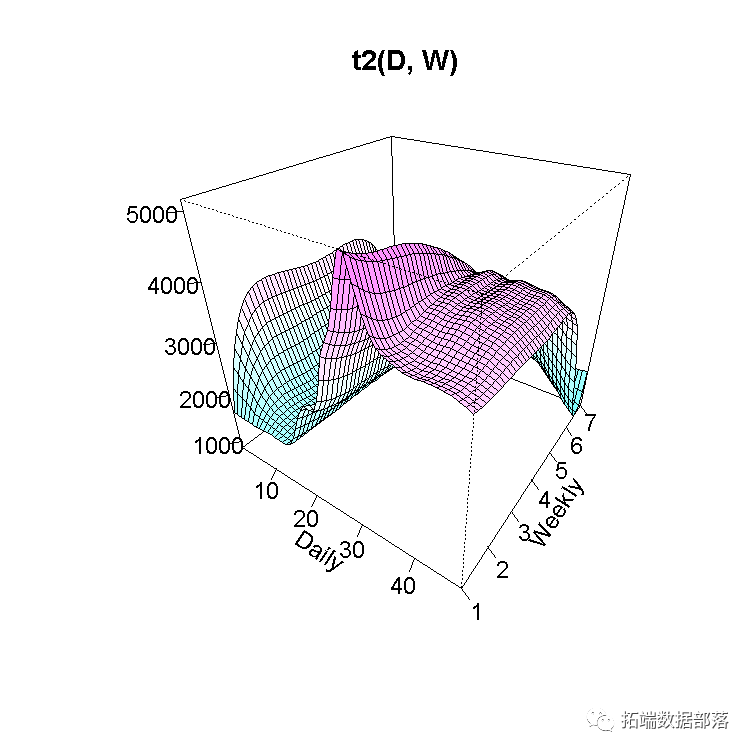​​

``````vis.gam(gam_6, main = "t2(D, W)", plot.type = "contour",
color = "terrain", contour.col = "black", lwd = 2)``````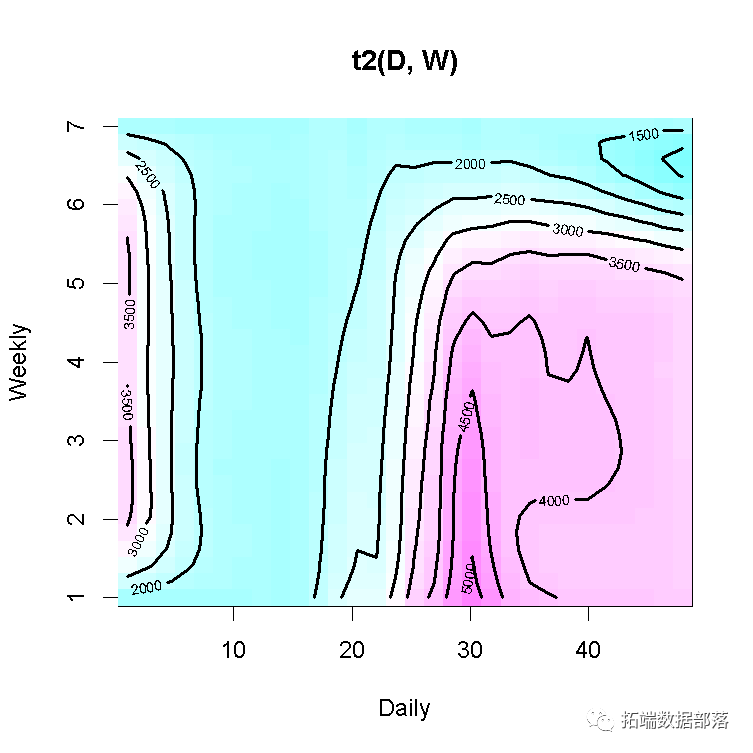​​

Kaizong Ye拓端研究室（TRL）的研究员。

​非常感谢您阅读本文，如需帮助请联系我们！QQ在线咨询

15121130882

0571-63341498

## 关注有关新文章的微信公众号

This will close in 0 seconds